Basics of Geometry
edHelper subscribers - Create a new printable

Number of Keys
 Select the number of different printables: 1 key 2 keys 3 keys 4 keys 5 keys

Number of Pages (for each key)
 Select the number of pages: 1 page 2 pages 3 pages 4 pages

Include an answer key (answer keys will be at the end of the printable)
High School Geometry
High School Geometry
Math

 Name _____________________________Date ___________________ (Key 1 - Answer ID # 0189294)
1.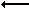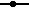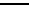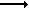E L J
The length of line segment EJ is 23. The length of line segment EL is 3x - 46. The length of line segment LJ is 4x - 57. What is the length of line segment EL?
2.   How many points does a line contain?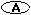three or more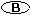an infinite number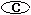one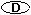at least one million
3.   Points that are all in one plane are ___________.collinearnoncoplanarnoncollinearcoplanar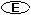None of the above
4.   Points that are not all in the same plane are ___________.collinearnoncoplanarcoplanarnoncollinearNone of the above
5.
 ST
represents a ___________.lengthsegmentrayline
6.   Given:
PH = 5 + 2x
DQ = 4x - 17PH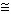DQ

What is the value of x?
7.   Two points on a number line have coordinates -32 and 42. What is the distance between the points?
8.QKQK

is an example of what property?reflexivesymmetrictransitive
9.OI
represents a ___________.segmentlengthlineray
10.   How many points do two intersecting lines have in common?onetwomore than one

Basics of Geometry
edHelper subscribers - Create a new printable

Number of Keys
 Select the number of different printables: 1 key 2 keys 3 keys 4 keys 5 keys

Number of Pages (for each key)
 Select the number of pages: 1 page 2 pages 3 pages 4 pages